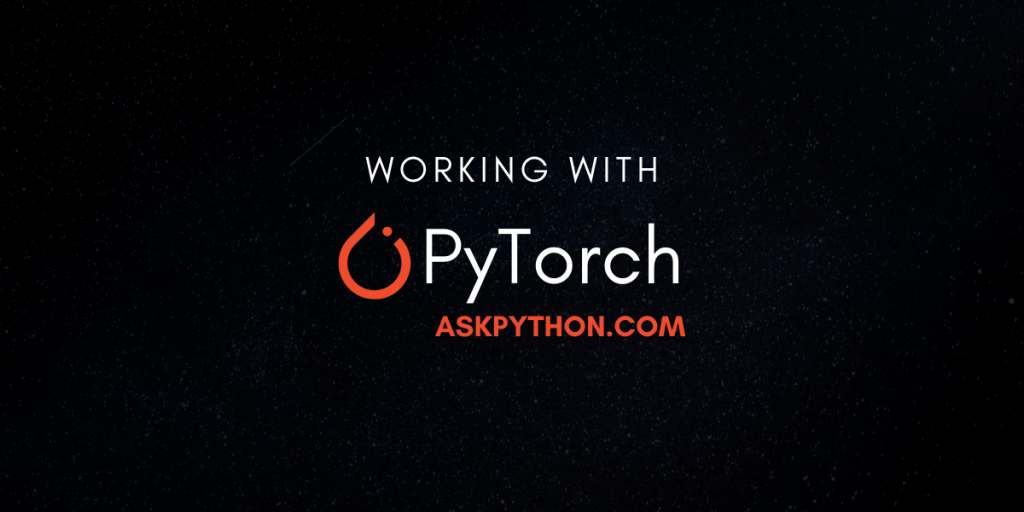# PyTorch – An Easy Beginner’s ReferenceMaking its debut a short four years ago, PyTorch is one of the modules that took the data science industry by storm.

Providing the users with well-documented code, tutorials, and examples to get started working with PyTorch, it was a huge hit in the eyes of data scientists and researchers alike.

The creators of PyTorch were also the ones who have worked on TorchVision and TorchText, both modules which were considered to be very useful in the fields of computer vision and natural language processing.

The PyTorch is a module that is mainly based on working with Tensors and Dynamic neural networks in Python, but, can extend to working with different areas as well.

In case you’re looking to work with PyTorch, we can help you get started right here!

It is also recommended to work with Anaconda for Data Science and Machine Learning, so, you might also want to look into that.

## Install PyTorch

The Official PyTorch website provides us with a simple interface to play around and retrieve the required installation command, based on your distribution and operating system.

In case you want to keep your normal environment separate from your Data Science environment, you should look into creating Virtual Environments.

Mess around with this for a bit, to pick an apt version for your local PyTorch library and we can go in, and work with PyTorch.

## Starting out with PyTorch

If you’ve installed PyTorch, great! We’re all set to start working with it now.

### 1.0 Tensors?

If you’ve ever worked with large matrices in Python, you probably use NumPy. This is because NumPy provides great support in working with multi-dimensional arrays allowing for working with various different operations on the n-dimensional arrays.

Well, PyTorch brings competition to the field with Tensor technology.

Tensors are in a sense multi-dimensional arrays, much like what NumPy provides. However, the difference lies in the fact that Tensors are pretty well supported when working with GPUs.

Google’s Tensorflow also operates on tensors to process and work with data.

So, how do we get started with Tensors and PyTorch? Let’s find out.

### 1.1. Importing Torch

We already know that working with any module would first require an import to include it in the script. As such, let’s do exactly that,

```# Importing torch to use in the script.
import torch
```

### 1.2. Creating Tensors

Creating Tensors, which are essentially matrices, using the `torch` module is pretty simple. Here are a few methods in order to initialize/create tensor objects.

```# Creating tensors with strict numbers
ten1 = torch.tensor(5)
ten2 = torch.tensor(8)
print(ten1, ten2)
# Output : tensor(5) tensor(8)

# Creating a matrix of zeros using the zeros function
ten3 = torch.zeros((3, 3))
print(ten3)
# tensor([[0., 0., 0.],
[0., 0., 0.],
[0., 0., 0.]])

# Creating a matrix of random numbers
ten4 = torch.randn(3, 3)
print(ten4)
# tensor([[-0.9685, 0.7256, 0.7601],
[-0.8853, 0.4048, -1.0896],
[0.6743, 1.5665, 0.2906]])
```

### 1.3. Basic Tensor operations

Tensors can be worked with, in many ways, much like a matrix created by the NumPy module.

We can work with basic numeric operations,

```firstten = torch.tensor(3)
secondten = torch.tensor(6)

print(firstten + secondten)
# Output : tensor(9)

# Subtraction of tensors
print(firstten - secondten)
# Output : tensor(-3)

# Multiplication of tensors
print(firstten * secondten)
# Output : tensor(18)

# Division of tensors
print(firstten / secondten)
# Output : tensor(0.5000)
```

### 1.4. Moving forward with Tensors

Tensors can be used for a lot more than simple operations which can be done with variables normally in Python.

They provide support for multiple operations to be performed on them, and are used as the variables normally in a lot of the operations in a PyTorch script.

With functions like matrix multiplication `torch.mm`, creating equal spaced tensors `torch.linspace`, and processing mathematical functions with math functions like `torch.sin(x)`.

It should come as no surprise that the functionality provided allows for deep computation with mathematical approaches embedded in them.

In case you wish to look into the working of tensors through examples, you might wish to look here.

## What’s Next?

Apart from working with the usual tensors, and the provided functions by default, it might be good to look into another module provided by PyTorch.

Tensors in itself is just a base after all. The true power lies in the application usage that the modules provide to use this medium for computation.

PyTorch provides us with modules designed for working with neural networks, called `torch.nn`.

The `torch.nn` modules contains a large variety of functions to help it perform operations based on neural networking like,

Working with these would be the next step in moving forward with PyTorch as your module for Data Science.

## Conclusion

PyTorch is a work in development, and yet provides functionality that is considered widely superior to a lot of Data Science modules.

There exist a large number of modules which are being created to be compatible with PyTorch and a large number of resources which help in working with them as well.

PyTorch is an Open Source project, and this means that you can work on the project and contribute to it’s future versions as well.

Here’s the GitHub link, and here’s to Open Source! Cheers!

Look into our other articles that will help you with your Data Science projects – Pandas, and Training and testing with sklearn.